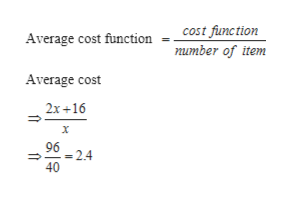A company can produce LCD digital alarm clocks at a cost of \$2 each while fixed costs are \$16. Therefore, the company's cost function is C(x) = 2x + 16.(a) Find the average cost function AC(x) = c(x)/xAC(x) =   (b) Find the marginal average cost function MAC(x).MAC(x) =   (c) Evaluate MAC(x) at x = 40. (Round your answer to the nearest cent.)

Question

A company can produce LCD digital alarm clocks at a cost of \$2 each while fixed costs are \$16. Therefore, the company's cost function is

C(x) = 2x + 16.
(a) Find the average cost function
AC(x) = c(x)/x
AC(x) =

(b) Find the marginal average cost function
MAC(x).

MAC(x) =

(c) Evaluate
MAC(x)
at
x = 40.
(Round your answer to the nearest cent.)
Step 1

Given cost function is fc= 2x+16

Where x is LCD digital alarm clock

Step 2

For x=40

Cost of 40 LCD digital alarm clock

Step 3

Average cost funct...help_outlineImage Transcriptionclosecost function Average cost function number of item Average cost 2x 16 96 2.4 40 fullscreen

Want to see the full answer?

See Solution

Want to see this answer and more?

Our solutions are written by experts, many with advanced degrees, and available 24/7

See Solution
Tagged in

Calculus